Latest Banking jobs   »

# Quantitative Aptitude Quiz For RBI Grade B Phase 1 2023 -12th April

Q1. Kapil got his salary increased by 15% while Dinesh got only 10% increment. Archu also got an increment of 20%. If ratio of earlier salary of Kapil, Dinesh and Archu is 3:4:5 respectively then find increased salary of Kapil will be what percent of that of Archu?
(a) 78%
(b) 57.5%
(c) 55.5%
(d) 58.5%
(e) 73.33%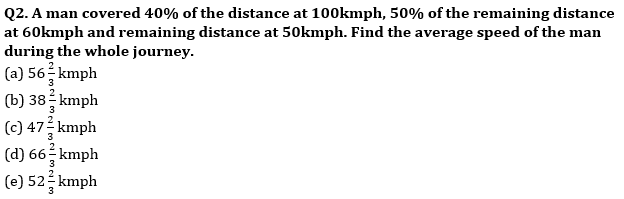Q3. A man invested Rs. X and Rs. 2X at 15% simple interest and at 8% compound interest (compounding annually) for two years respectively. Difference between simple interest and compound interest received after two years is Rs.820. Find the value of X.
(a) Rs.25000
(b) Rs.30600
(c) Rs.28600
(d) Rs.22200
(e) Rs.26200
Q4. A and B have equal amount, while A invested his amount on C.I. for two years at the rate of 10% p.a. and B invested 2/3 rd of his amount at the rate of R% p.a. on simple interest and remaining amount at the rate of 6.5% p.a. on also simple interest. If interest received by both at the end of two years are equal, then find the value of (R + 2.5) %?
(a) 12%
(b) 15%
(c) 17.5%
(d) 22.5%
(e) 12.5%

Q5. A invested Rs. X in a scheme. After 6 months, B joined with Rs. 4000 more than that of A. After a year, ratio of profit of B to the total profit was 3: 7. Find the value of X.
(a) 4000
(b) 8000
(c) 1600
(d) 6000
(e) 10000
Q6. In first mixture, quantity of milk was 6 lit more than that of water. After adding 40 ltr of second mixture (having ratio of water to milk 3: 5) in the first mixture, ratio of water to milk in the final mixture becomes 9: 13. Find the total quantity of water in the final mixture.
(a) 27 ltr
(b) 31.5 ltr
(c) 36 ltr
(d) 45 ltr
(e) None of these
Q7. In 100 lit mixture of milk and water, milk is 68 lit more than that of water. When ‘a’ lit of milk is taken out and (a+15) lit of water is added, milk becomes 50% more than that of water. Find value of ‘a’?
(a) 20
(b) 15
(c) 18
(d) 12
(e) 16
Q8. Two varieties of wheat is mixed in the ratio of 2:3 by weight. The price of the mixture is Rs.12/kg and the price of the variety having lower weight is Rs 10/kg. Find the price per kg of the other variety. (in Rs)
(a) 35/3
(b)50/3
(c) 38/3
(d)47/3
(e)40/3
Q9. A, B and C can do a work in 12,15 and 20 days respectively. All three started together but C left in between before completion of work. The remaining work was completed by A and B and the total work was completed in 6 days. Find after how many days C left the work?
(a)2
(b)3
(c)4
(d)1
(e)5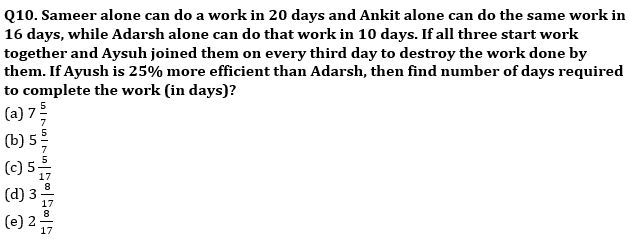Q11. Speed of a train is 1/3 rd of speed of plane, while speed of train is 1.5 times more than speed of a car. If car can travel 800 km in 12.5 hours, then find difference between distance cover by train in 7.5 hour and plane in 4.5 hours (in km)?
(a) 960
(b) 720
(c) 840
(d) 1080
(e) 1120

Q12. A person covered 9 km at 3 km/hr, 15 km at 5 km/hr and 30 km at 10 km/hr. then find the average speed of person in covering the entire distance?
(a) 5 km/hr
(b) 6 km/hr
(c) 7 km/hr
(d) 8 km/hr
(e) 7.5 km/hr
Q13. A train travels 60% faster than a car. Both start from point A at the same time and reach point B, which is 160 km away at the same time. If on the way the train stop for 20 minutes at station, then find the speed (in km/hr) of the train?
(a) 144
(b) 168
(c) 198
(d) 288
(e) 248

Q14. 45% of first number is equal to 60% of second number. If average of both the numbers is 10 less than the first number, then find 80% of second number?
(a) 64
(b) 40
(c) 48
(d) 56
(e) 80
Q15. The sum of Ravi’s and Shivam’s age 4 years ago was 80 years. Ravi’s age 14 years ago was equal to Shivam’s age 4 years ago, then find the present age of Ravi ? (in years)
(a) 39
(b) 49
(c) 40
(d) 47
(e) 55

Solutions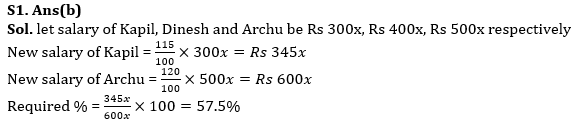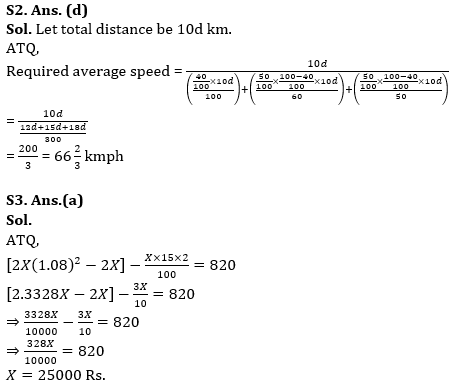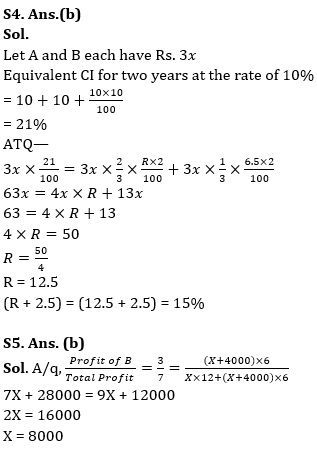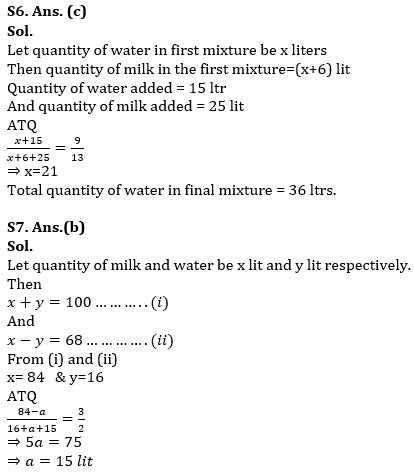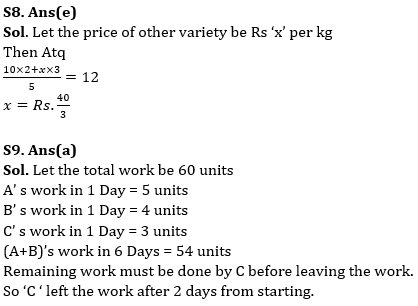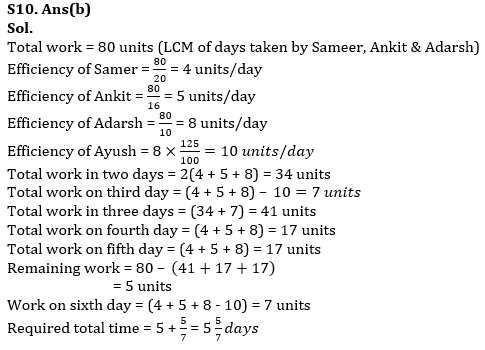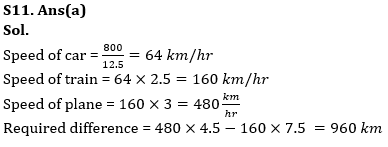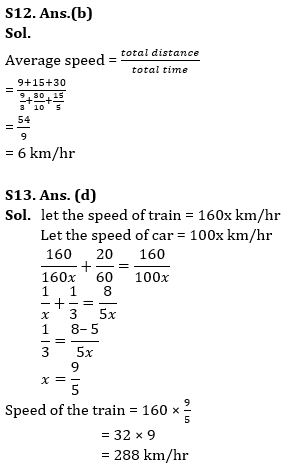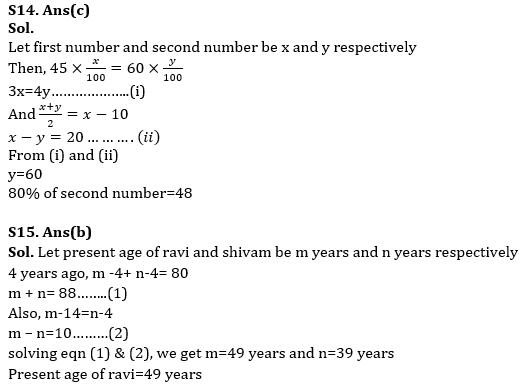## FAQs

### How many sections are there in the RBI Grade B Phase 1 Exam?

There are 4 sections in the RBI Grade B Phase 1 Exam i.e. English Language, General Awareness, Quantitative Aptitude & Reasoning.

#### Congratulations!Union Budget 2023-24: Free PDF